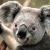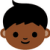# stream.js ：一个新的JavaScript数据结构热

stream.js 是一个很小、完全独立的Javascript类库，它为你提供了一个新的Javascript数据结构：streams

`<script src='stream-min.js'></script> `

## streams是什么？

Streams 是一个操作简单的数据结构，很像数组或链接表，但附加了一些非凡的能力。

## 入门

Stream 是一种容器。它能容纳元素。你可以使用 `Stream.make` 来让一个stream加载一些元素。只需要把想要的元素当成参数传进去：

```var s = Stream.make( 10, 20, 30 );
// s is now a stream containing 10, 20, and 30  ```

```    var s = Stream.make( 10, 20, 30 );
console.log( s.length() );  // outputs 3
console.log( s.head() );    // outputs 10
console.log( s.item( 0 ) ); // exactly equivalent to the line above
console.log( s.item( 1 ) ); // outputs 20
console.log( s.item( 2 ) ); // outputs 30  ```

```var s = Stream.make( 10, 20, 30 );
var t = s.tail();         // returns the stream that contains two items: 20 and 30
console.log( t.head() );  // outputs 20
var u = t.tail();         // returns the stream that contains one item: 30
console.log( u.head() );  // outputs 30
var v = u.tail();         // returns the empty stream
console.log( v.empty() ); // prints true  ```

```    var s = Stream.make( 10, 20, 30 );
while ( !s.empty() ) {
s = s.tail();
}  ```

## 用它们还能做什么？

```var s = Stream.range( 10, 20 );
s.print(); // prints the numbers from 10 to 20  ```

```function doubleNumber( x ) {
return 2 * x;
}

var numbers = Stream.range( 10, 15 );
numbers.print(); // prints 10, 11, 12, 13, 14, 15
var doubles = numbers.map( doubleNumber );
doubles.print(); // prints 20, 22, 24, 26, 28, 30```

```function checkIfOdd( x ) {
if ( x % 2 == 0 ) {
// even number
return false;
}
else {
// odd number
return true;
}
}
var numbers = Stream.range( 10, 15 );
numbers.print();  // prints 10, 11, 12, 13, 14, 15
var onlyOdds = numbers.filter( checkIfOdd );
onlyOdds.print(); // prints 11, 13, 15```

```function printItem( x ) {
console.log( 'The element is: ' + x );
}
var numbers = Stream.range( 10, 12 );
// prints:
// The element is: 10
// The element is: 11
// The element is: 12
numbers.walk( printItem );```

```var numbers = Stream.range( 10, 100 ); // numbers 10...100
var fewerNumbers = numbers.take( 10 ); // numbers 10...19
fewerNumbers.print();```

```var numbers = Stream.range( 1, 3 );
var multiplesOfTen = numbers.scale( 10 );
multiplesOfTen.print(); // prints 10, 20, 30
numbers.add( multiplesOfTen ).print(); // prints 11, 22, 33```

## 这需要用上你无穷多的内存/时间/处理能力吗？

```var naturalNumbers = Stream.range(); // returns the stream containing all natural numbers from 1 and up
var oneToTen = naturalNumbers.take( 10 ); // returns the stream containing the numbers 1...10
oneToTen.print();```

## 你在骗人

```var naturalNumbers = Stream.range(); // naturalNumbers is now 1, 2, 3, ...
var evenNumbers = naturalNumbers.map( function ( x ) {
return 2 * x;
} ); // evenNumbers is now 2, 4, 6, ...
var oddNumbers = naturalNumbers.filter( function ( x ) {
return x % 2 != 0;
} ); // oddNumbers is now 1, 3, 5, ...
evenNumbers.take( 3 ).print(); // prints 2, 4, 6
oddNumbers.take( 3 ).print(); // prints 1, 3, 5```

```var s = new Stream( 10, function () {
return new Stream();
} );
// the head of the s stream is 10; the tail of the s stream is the empty stream
s.print(); // prints 10
var t = new Stream( 10, function () {
return new Stream( 20, function () {
return new Stream( 30, function () {
return new Stream();
} );
} );
} );
// the head of the t stream is 10; its tail has a head which is 20 and a tail which
// has a head which is 30 and a tail which is the empty stream.
t.print(); // prints 10, 20, 30```

```function ones() {
return new Stream(
// the first element of the stream of ones is 1...
1,
// and the rest of the elements of this stream are given by calling the function ones() (this same function!)
ones
);
}

var s = ones();      // now s contains 1, 1, 1, 1, ...
s.take( 3 ).print(); // prints 1, 1, 1```

```function ones() {
return new Stream( 1, ones );
}
function naturalNumbers() {
return new Stream(
// the natural numbers are the stream whose first element is 1...
1,
function () {
// and the rest are the natural numbers all incremented by one
// which is obtained by adding the stream of natural numbers...
// 1, 2, 3, 4, 5, ...
// to the infinite stream of ones...
// 1, 1, 1, 1, 1, ...
// yielding...
// 2, 3, 4, 5, 6, ...
// which indeed are the REST of the natural numbers after one
}
);
}
naturalNumbers().take( 5 ).print(); // prints 1, 2, 3, 4, 5```

```function sieve( s ) {
return new Stream( h, function () {
return sieve( s.tail().filter( function( x ) {
return x % h != 0;
} ) );
} );
}
sieve( Stream.range( 2 ) ).take( 10 ).print();```

## 感谢你的阅读！

11~~~

00
l
beautiful